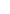## Oscillators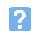1. The circuit shown below is: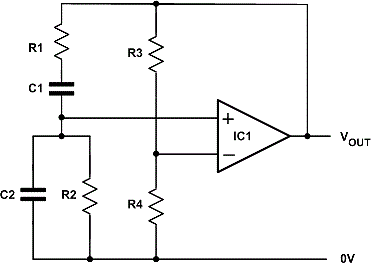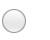a) an astable multivibratorb) a Wien bridge oscillatorc) a triangle wave generatord) a ladder network oscillator.2. In the circuit shown in Question 1, the frequency of oscillation is determined by:a) R3 and R4b) R1, C1 and R2, C2c) R3, C1 and R4, C2d) R1, R3 and R2, R4.3. In order to obtain oscillation in the circuit shown:a) R1 > 2 x R2b) R3 > 2 x R4c) R2 > 2 x R1d) R4 > 2 x R3.4. The circuit shown below is: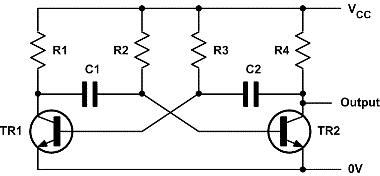a) an astable multivibratorb) a Wien bridge oscillatorc) a triangle wave generatord) a ladder network oscillator.5. In the circuit shown, the frequency of oscillation is determined by:a) R3 and R4b) R1, C1 and R4, C2c) R2, C1 and R3, C2d) R1, R3 and R2, R4.6. The output waveform produced by the circuit shown will be:a) a ramp waveb) a sine wavec) a square waved) a triangle wave.7. The component shown in the photo below is: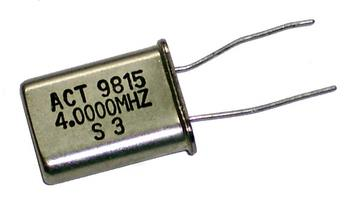a) a capacitorb) a transformerc) a quartz crystald) a semiconductor diode.8. A typical application for the component shown is:a) generating white noise for use when testing an amplifierb) acting as a source of voltage for a low-power oscillatorc) stabilising the frequency of a high-frequency oscillatord) providing additional voltage gain required for oscillation.9. The output waveform produced by the circuit shown will be: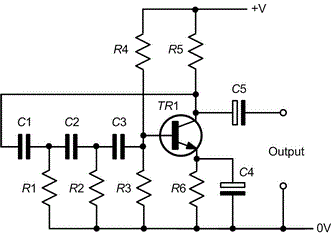a) a ramp waveb) a sine wavec) a square waved) a triangle wave.10. The network comprising C1, R1, C2, R2, C3 and R3/R4 provides a phase shift of:a) 90 degreesb) 180 degreesc) 270 degreesd) 360 degrees.
This is more feedback!
This is the feedback!